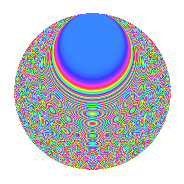# Properties

 Label 8026.2.aLevel 8026 Weight 2 Character orbit a Rep. character $$\chi_{8026}(1,\cdot)$$ Character field $$\Q$$ Dimension 334 Newform subspaces 4 Sturm bound 2007 Trace bound 1

# Related objects

## Defining parameters

 Level: $$N$$ = $$8026 = 2 \cdot 4013$$ Weight: $$k$$ = $$2$$ Character orbit: $$[\chi]$$ = 8026.a (trivial) Character field: $$\Q$$ Newform subspaces: $$4$$ Sturm bound: $$2007$$ Trace bound: $$1$$

## Dimensions

The following table gives the dimensions of various subspaces of $$M_{2}(\Gamma_0(8026))$$.

Total New Old
Modular forms 1005 334 671
Cusp forms 1002 334 668
Eisenstein series 3 0 3

The following table gives the dimensions of the cuspidal new subspaces with specified eigenvalues for the Atkin-Lehner operators and the Fricke involution.

$$2$$$$4013$$FrickeDim.
$$+$$$$+$$$$+$$$$81$$
$$+$$$$-$$$$-$$$$86$$
$$-$$$$+$$$$-$$$$96$$
$$-$$$$-$$$$+$$$$71$$
Plus space$$+$$$$152$$
Minus space$$-$$$$182$$

## Trace form

 $$334q + 334q^{4} + 4q^{5} - 2q^{6} + 328q^{9} + O(q^{10})$$ $$334q + 334q^{4} + 4q^{5} - 2q^{6} + 328q^{9} + 6q^{10} + 2q^{11} - 2q^{13} + 334q^{16} + 16q^{17} - 10q^{19} + 4q^{20} - 4q^{22} + 12q^{23} - 2q^{24} + 340q^{25} + 4q^{26} - 24q^{30} - 4q^{31} - 20q^{33} - 8q^{34} + 4q^{35} + 328q^{36} - 4q^{37} - 16q^{38} - 56q^{39} + 6q^{40} + 12q^{41} - 6q^{43} + 2q^{44} + 20q^{45} - 8q^{46} + 16q^{47} + 346q^{49} + 8q^{50} - 16q^{51} - 2q^{52} + 10q^{53} - 20q^{54} + 32q^{55} - 20q^{57} + 10q^{58} - 6q^{59} - 22q^{61} + 8q^{62} - 44q^{63} + 334q^{64} - 4q^{65} - 16q^{66} - 14q^{67} + 16q^{68} - 28q^{69} + 20q^{70} + 4q^{73} + 10q^{74} - 28q^{75} - 10q^{76} + 16q^{77} + 20q^{78} - 20q^{79} + 4q^{80} + 294q^{81} + 16q^{82} - 2q^{83} - 4q^{85} - 8q^{86} - 4q^{87} - 4q^{88} + 4q^{89} + 30q^{90} + 4q^{91} + 12q^{92} + 8q^{93} + 4q^{94} - 28q^{95} - 2q^{96} - 4q^{97} + 46q^{99} + O(q^{100})$$

## Decomposition of $$S_{2}^{\mathrm{new}}(\Gamma_0(8026))$$ into newform subspaces

Label Dim. $$A$$ Field CM Traces A-L signs $q$-expansion
$$a_2$$ $$a_3$$ $$a_5$$ $$a_7$$ 2 4013
8026.2.a.a $$71$$ $$64.088$$ None $$71$$ $$-9$$ $$-34$$ $$-19$$ $$-$$ $$-$$
8026.2.a.b $$81$$ $$64.088$$ None $$-81$$ $$-10$$ $$-26$$ $$3$$ $$+$$ $$+$$
8026.2.a.c $$86$$ $$64.088$$ None $$-86$$ $$11$$ $$25$$ $$-3$$ $$+$$ $$-$$
8026.2.a.d $$96$$ $$64.088$$ None $$96$$ $$8$$ $$39$$ $$19$$ $$-$$ $$+$$

## Decomposition of $$S_{2}^{\mathrm{old}}(\Gamma_0(8026))$$ into lower level spaces

$$S_{2}^{\mathrm{old}}(\Gamma_0(8026)) \cong$$ $$S_{2}^{\mathrm{new}}(\Gamma_0(4013))$$$$^{\oplus 2}$$

## Hecke Characteristic Polynomials

There are no characteristic polynomials of Hecke operators in the database